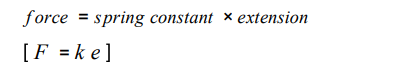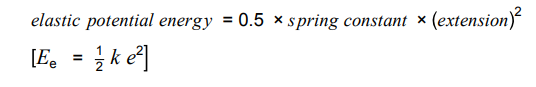# Resources

The specification is a good place to start. Page 47 for this one. https://filestore.aqa.org.uk/resources/physics/specifications/AQA-8463-SP-2016.PDF

The BBC Bitesize website is also helpful for diagrams and graphs and clear explanations. https://www.bbc.co.uk/bitesize/guides/z9hk3k7/revision/1

# Questions

1. Draw a force diagram showing two forces compressing, bending and stretching a spring.
2. Why do we need more than one force to change the shape of an object e.g stretching, compressing or bending the object?
3. What is the difference between elastic deformation and inelastic deformation caused by stretching forces?
4. Springs: What is the mathematical relationship between the force applied on a spring and extension?
5. Make sure that you learn this equation.6. What are the units of force?
7. What are the units of extension?
8. What are the units of spring constant?
9. Can you explain what the spring constant tells you about?
10. Can the ‘e’ in the equation also be how much the object has been compressed by?
11. Copy the paragraph below filling in the gaps. The specification will help you with this.

A force that stretches (or compresses) a spring does _________ and ________________ is stored in the spring. Provided the spring is not ____________ deformed, the work done on the spring and the elastic potential energy stored are_________.

1. What is the difference between a linear and non-linear relationship between force and extension?
2. How can the spring constant be calculated from a force-extension graph? Force on the y axis and extension on the x-axis.
3. Can you rearrange this equation?4. Rearrange the equation for k.
5. Rearrange the equation for e. This involves a square root.